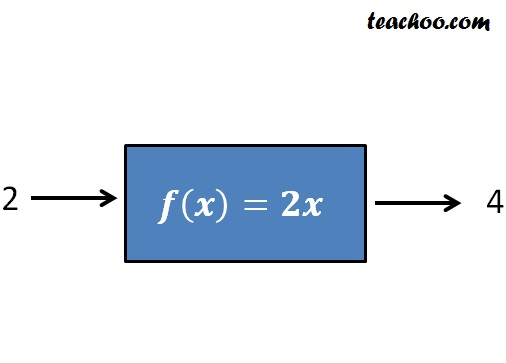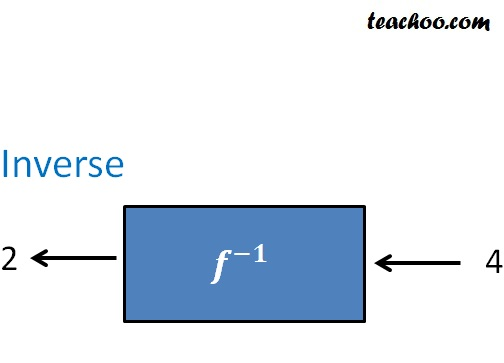Finding Inverse

Chapter 1 Class 12 Relation and Functions
Concept wise

Suppose we have a function

f(x) = 2x

So, if we input 2, we get 4 backIn Inverse of f ,

the opposite happens

i.e. we input 4 and we get 2## How to find the inverse?

f(x) = 2x

We put f(x) = y and find x in terms of y

y = 2x

y/2 = x

x = y/2

∴ f -1 (y) = y/2

Now,

f(f -1 (x)) will always give back x

i.e. f(f -1 (x)) is an identity function

Let’s check

f -1 (y) = y/2

So, f -1 (x) = x/2

f(f -1 (x)) = f(x/2)

= 2 (x/2)

= x

Similarly,

f -1 (f(x)) will always give back x

i.e. f -1 (f(x)) is an identity function

Let’s check

f -1 (f(x)) = f -1 (2x)

= 2x/2

= x

Thus,

f(f -1 (x)) and f -1 (f(x)) are identity functions .

Also, function will have inverse only when it is one-one and onto.

Let's next see how to check if function has inverse.

Learn in your speed, with individual attention - Teachoo Maths 1-on-1 Class